Elliptic cohomology

A term first introduced in 1986 by P.S. Landweber, D.C. Ravenel and R.E. Stong (cf. [a2] and [a3]) to designate a cohomology theory obtained by tensoring oriented cobordism theory with a ring of modular forms in characteristic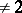. In the 1990{}s, numerous publications devoted to elliptic cohomology have appeared. On the one hand, they connect elliptic cohomology to other generalized cohomology theories, most notably to Morava-theories (cf. also-theory), on the other hand they attempt to give a geometric interpretation of elliptic cohomology. Despite a multitude of very interesting results, there seems to be no agreement on what exactly is elliptic cohomology. The situation is, however, quite well-understood outside the prime number.

Landweber–Ravenel–Stong theory.

Let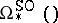be oriented bordism theory with coefficient ring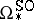, and let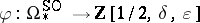be the level-elliptic genus (cf. Elliptic genera) with logarithm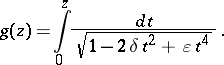The ringis the ring of level-modular forms in characteristic(cf. also Modular form) and can be viewed, via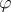, as an-module.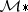has a natural grading, for which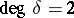,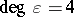.

Letbe any homogeneous element of positive degree. Then the functor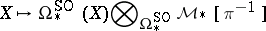(a1)

is a periodic homology theory (elliptic homology) with coefficient ring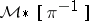.

A similar construction using oriented cohomology leads to a multiplicative periodic cohomology theory (elliptic cohomology). The proof of this theorem was first given by Landweber, Ravenel and Stong under the assumption thatis one of the factors of, and was based on the one hand on Landweber's exact functor theorem, and on the other hand on interesting congruences for Legendre polynomials. The general form stated above is due to J. Franke [a1], who showed that the exactness of the functor (a1) is a consequence of the Deuring–Eichler theorem, saying that the height of the formal group of an elliptic curve in positive characteristic is alwaysor.